# OECD Trust in government data analysis 5 - Simple linear regression using R - Trust in governance and per capita GDP, log(per capita GDP)Photo by Annie Spratt on Unsplash

This post is following of the above post.

5. Independent variable = capi, by year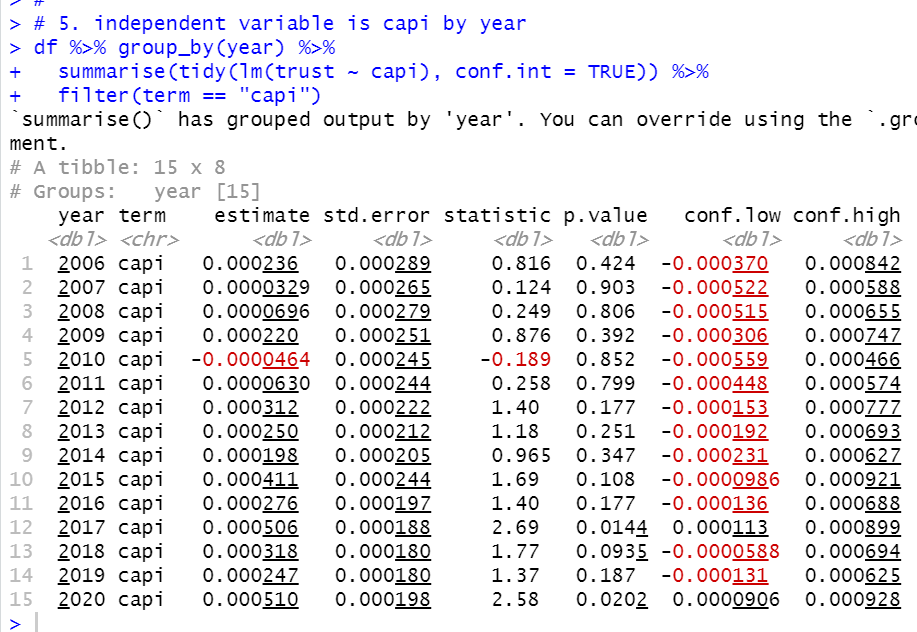Almost year except for 2010 have positive coefficientt. But only 2017 is statisticaly significant.

6. Independent variable = capi by iso.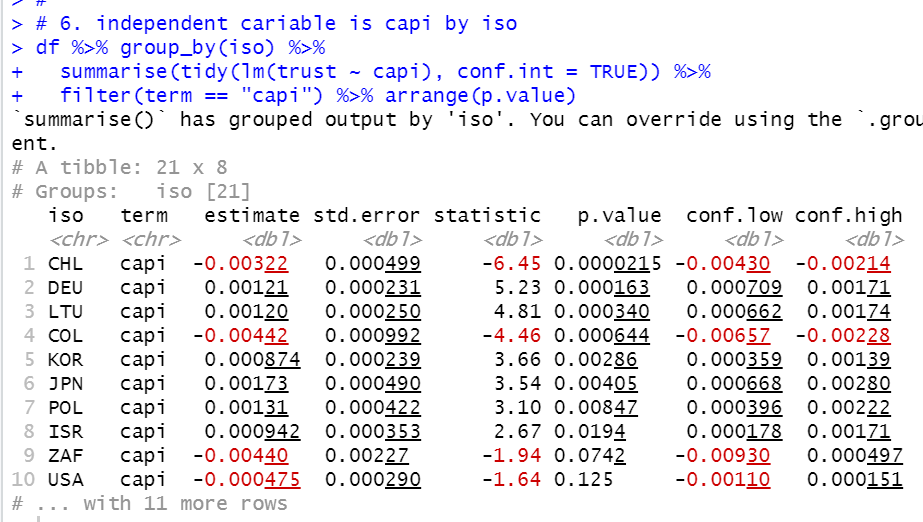Some countries has less than 0.05 p.value. CHL and COL have negative sign corfficients with statistically significant. There are only 8 iso-s which have less than 0.05 p.value.

7. Independent variable = l_capi by year.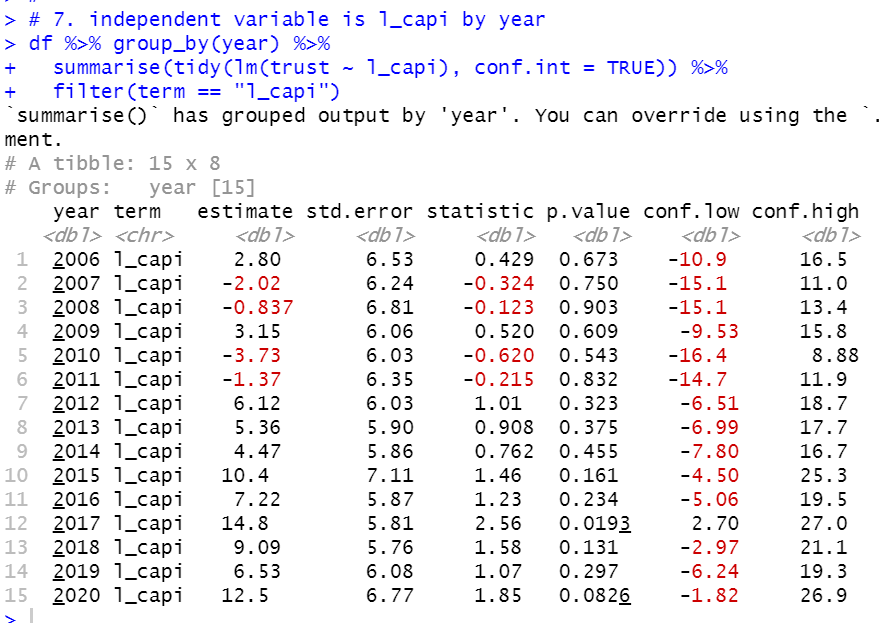2007, 2008, 2010 and 2011 have negative sign coefficient but they are not statistically significant. 2017 has only statistically significant.

8. Independent variable = l_capi by iso.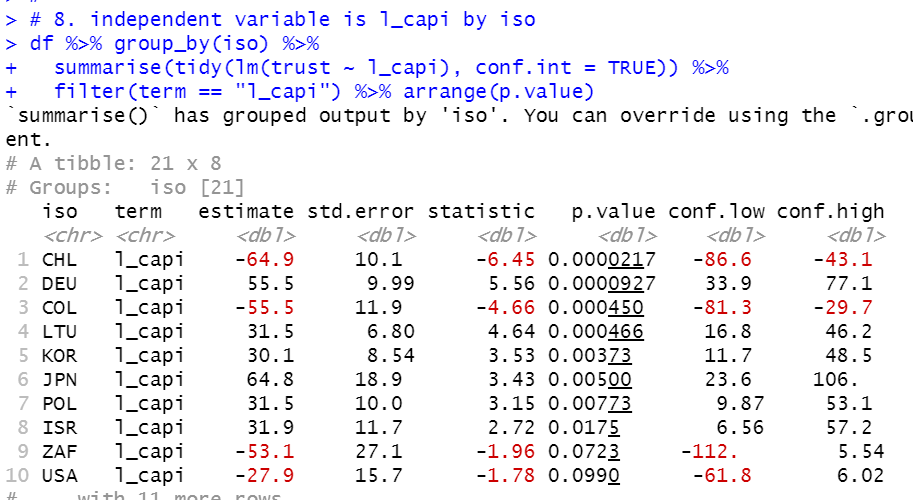Again, CHL and COL have negative coefficient and there are only 8 iso-s which have less than 0.05 p.value.

Let's make a scatter plot of CHL and COL only and DEU, LTU, KOR, JPN, POL, ISR only.

First, I made indexs for the both.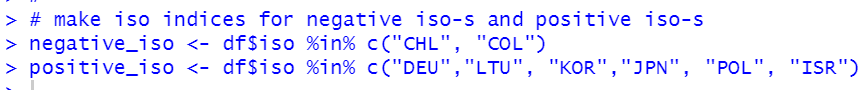Let's check those indeces will work fine.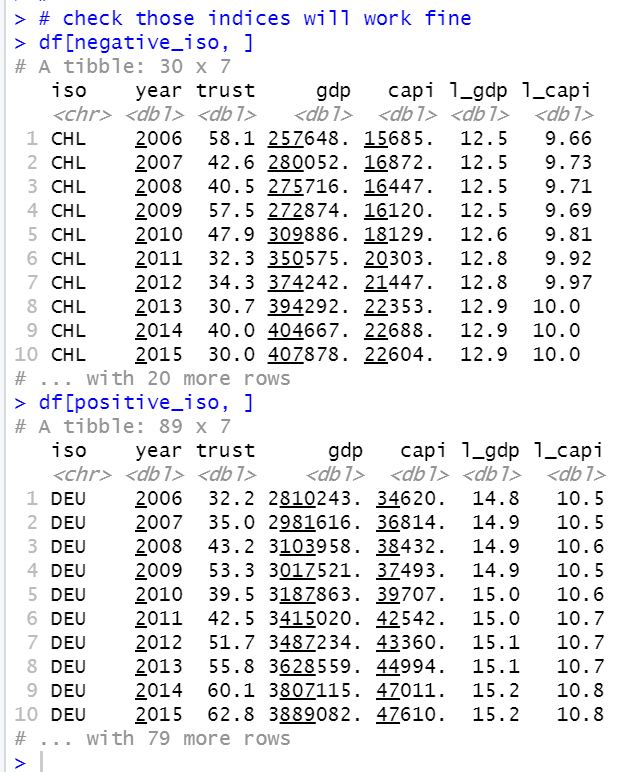All right. the both indices will do.

Let's make scatter plots.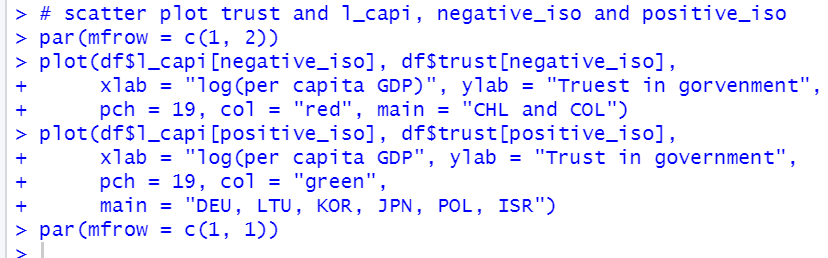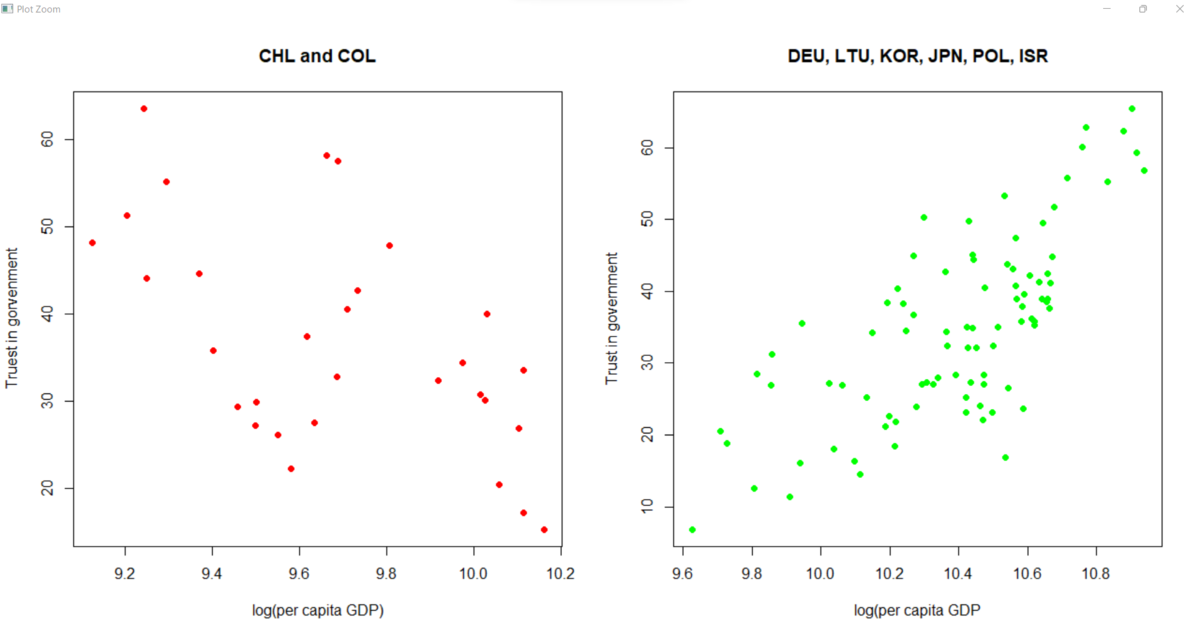Good.
Next, do linear regression for negative iso and positive iso.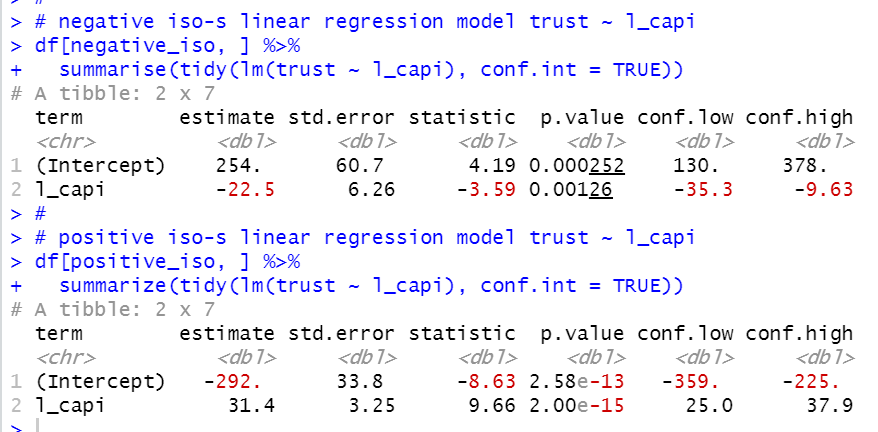That' s it. Thank you!

Next post is